# How to Find the Slope of a Line on an Excel Graph?

• Last Updated : 18 Jul, 2021

In this article, we will look into how to calculate the slope of a line in an Excel graph.

Slope of a line basically determines two parameters :

Attention reader! Don’t stop learning now. If you are an Excel beginner (or an intermediate) and want to learn Excel, Geeksforgeeks brings the perfect course for you to start, Diving Into Excel

1. The direction of a line.
2.  The steepness of a line (rise or fall).

It is generally denoted by the letter “m”. The equation of a line is given by the expression :Where,

• m: Slope
• c: Intercept

The mathematical formula for the slope of a line is given by the ratio of rise and run and in geometry, it is denoted using tan theta.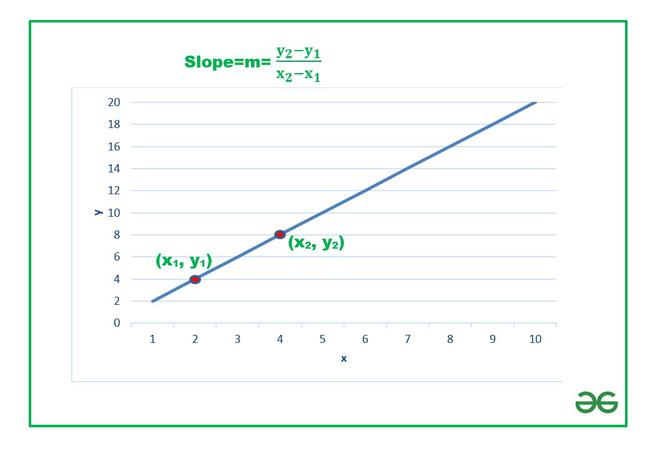In this article we are going to discuss various methods on how to find the slope of a line in Excel using a few examples.

Example 1 : Consider the dataset having x and y coordinates of a particle moving in 2-D plane.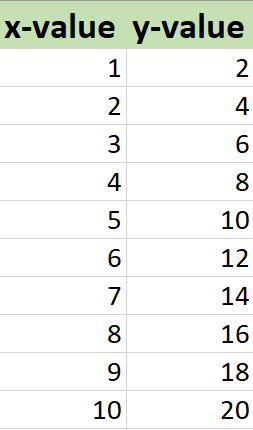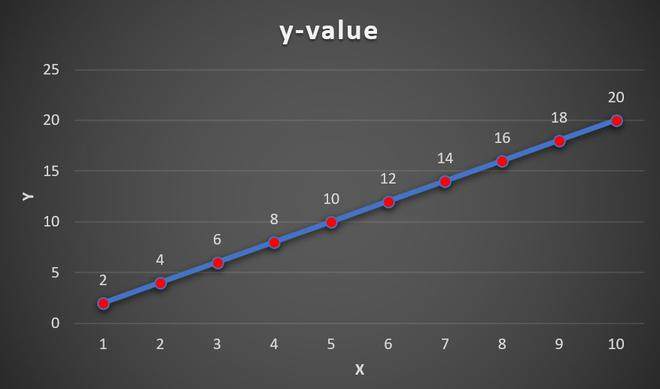Line Graph

## Finding Slope:

There are three methods :

1. By using the Excel in-built function SLOPE. The syntax is :

```=SLOPE(known_ys,knownx_s)

known_ys : An array of numeric data points which are dependent. These are dependent on value of horizontal axis.
known_xs : An array of numeric data points which are independent.```

2. By using the slope formula as discussed.

The steps are :

• From the data set take any pair of points.
• The points are (x1, y1) and (x2, y2).
• Use the formula and “-“,”/” operators to find the slope, m.

3. By plotting a trendline on the line graph and find its equation. From the equation of the trendline we can easily get the slope.

### Method 1: Using Slope function

Here, Y-axis array is stored in B column. The array ranges from B2 to B11.

The X-axis array is stored in A column of the Excel sheet. The array ranges from A2 to A11.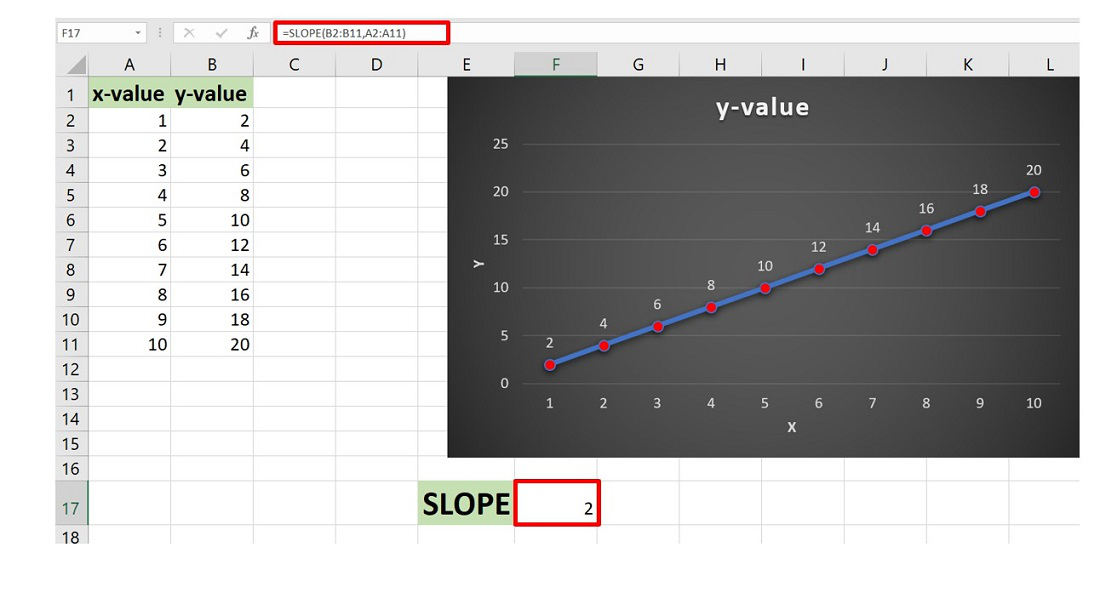### Method 2: Using Slope formula

Consider the points (1,2) and (2,4). Here, y1=2 and stored in the location B2 and y2=4 stored in location B3 and x1=1 stored in the location A2 and x2=2 stored in the location A3 of the worksheet.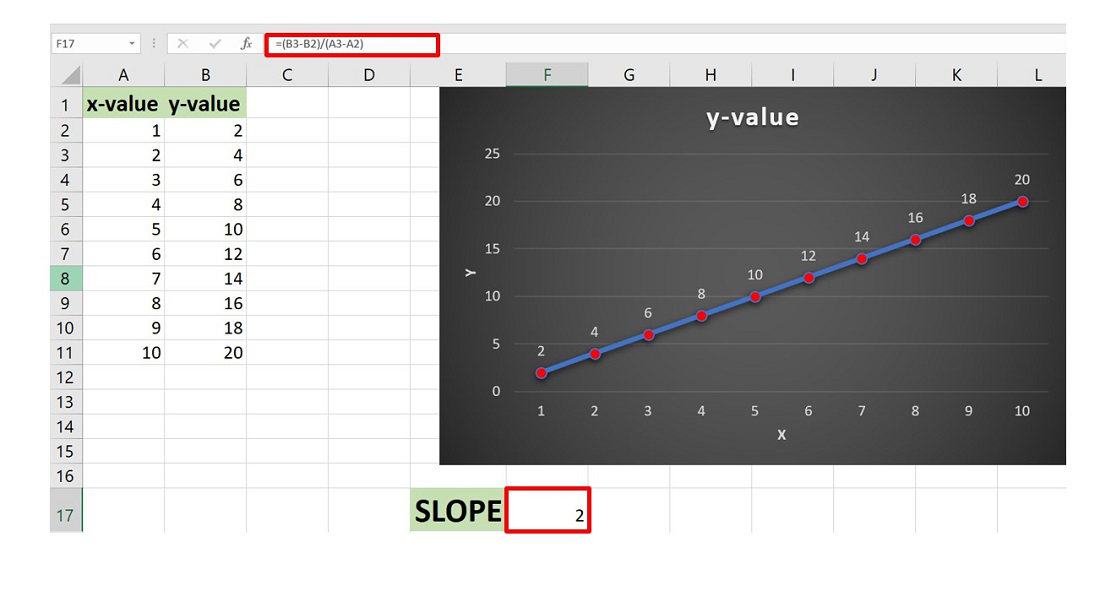### Method 3:

The steps are :

• Plot the line graph by selecting the dataset and then go to the Insert Tab and then click on Insert Line or Area Chart.
• The line chart is plotted.
• Now select the chart and then click on the “+” button in the top right corner of the Chart.
• The Chart Elements dialog box appears. In this check the Trendline option. This will add the trendline to the existing line graph.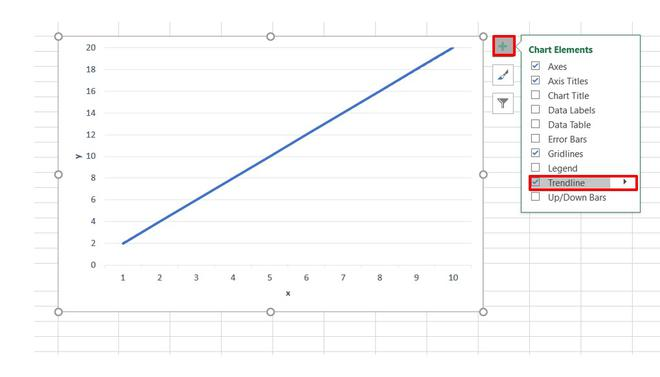• Now select the Trendline in the chart and right-click on it and then click on Format Trendline.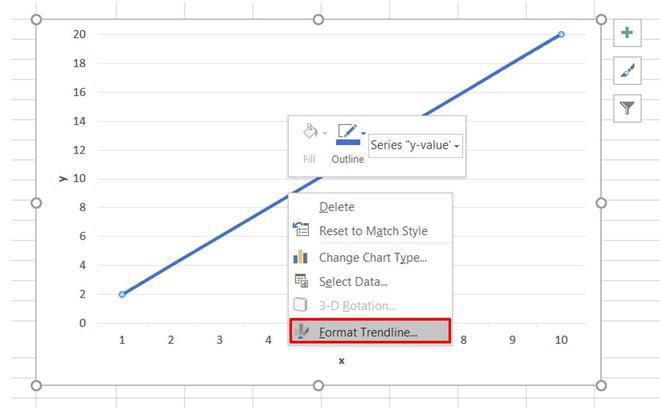• The Format Trendline dialog box opens. By default, the trendline will be linear.
• Now, check the box “Display Equation on the chart”.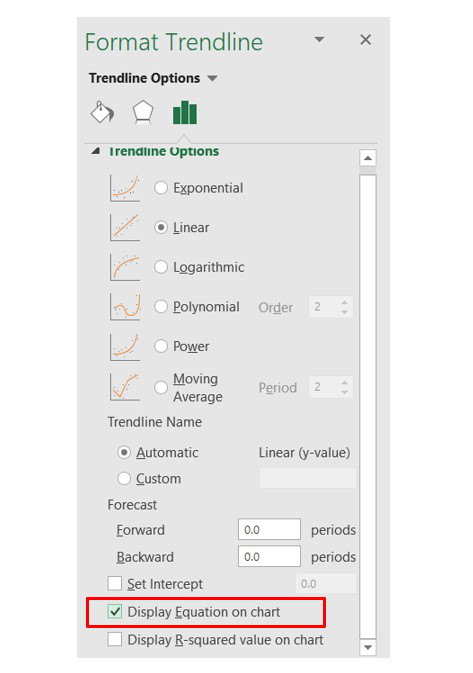• This will add the equation of the line on the chart.

The equation of the line is

y=2x

By comparing with the general equation y = mx + c, we get

m=2, c=0

The slope is 2 for the given line.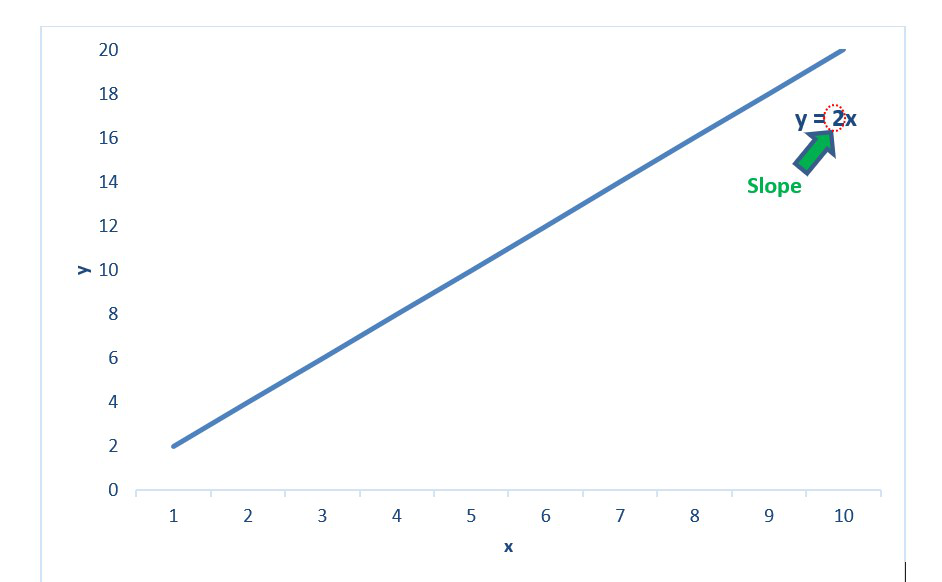Example 2: Consider the dataset shown below :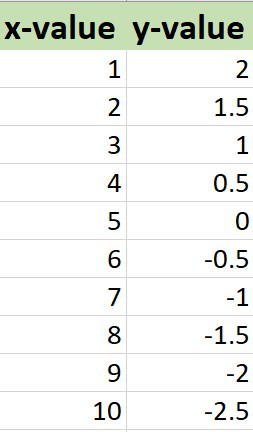Similarly, by using the trendline and its equation you can easily find the slope of the line is -0.5.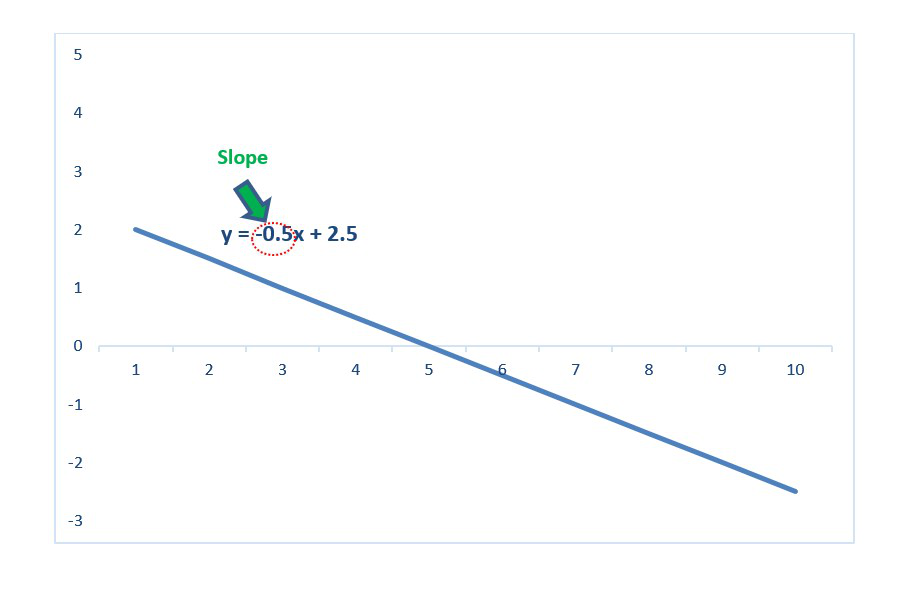My Personal Notes arrow_drop_up